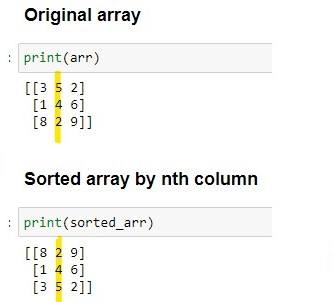# Sorting Arrays by nth Column

Sorting an array by the nth column using NumPy is a common task in data analysis and scientific computing. It involves rearranging the rows of the array based on the values in a specific column. The nth column refers to the column at index n-1, where n is the position of the column in the array.NumPy provides a lot of different convenient functionalities, which are listed below:

#### 1. Using “np.lexsort()”:

The code creates a 2D NumPy array called “arr”. It then sorts the array based on the second column (index 1) using `np.lexsort()`. The resulting sorted array is stored in “sorted_arr”, and it is printed to the console. The column to sort by is defined using the variable “n”.

• You can also use `np.lexsort()` and sort the array by multiple columns. The example below creates a 2D NumPy array called “arr”. It then sorts the array based on the second column (index 1), and then by the first column (index 0) using `np.lexsort()`. The resulting sorted array is stored in “sorted_arr”, and it is printed to the console.

Example:

#### 2. Using “np.argsort()” with descending order:

The code creates a 2D NumPy array called “arr”. It then sorts the array based on the second column (index 1) in descending order using `np.argsort(-arr[:,1])`. The resulting sorted array is stored in “sorted_arr”, and it is printed to the console. Note that `np.argsort()` sorts the array in ascending order by default. If you want to sort in descending order, you can use negative indexing.